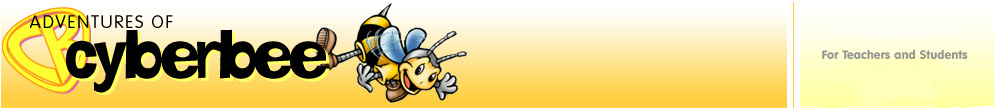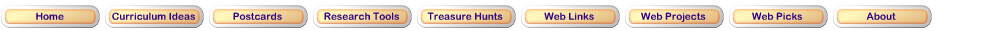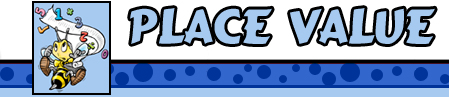Number and Operations Standard

Understand numbers, ways of representing numbers, relationships among numbers, and number systems
• understand the place-value structure of the base-ten number system and be able to represent and compare whole numbers and decimals;
• recognize equivalent representations for the same number and generate them by decomposing and composing numbers;

Step 1View this video How to Teach Comparisons from Expert Village.Step 2

Practice your Place Value skills by visiting these Websites with loads of activities.AAA Math Write the number that corresponds to the words.AAA Math Write the number for the expanded form.AAA Math Write the number that corresponds to the words.AAA Math Match the number to the place values.Quia Match each number to its expanded form.Quia Try to uncover matching pairs of cards. Match each number written in standard form with its correct corresponding number written in expanded form.Quia Click on the flashcard to flip it over. Match each number written in standard form with its correct corresponding number written in expanded form.E-Lab Use a place-value chart to find the value of each digit in a number.Funbrain Funbrain will show you a number. Click on the correct place value to uncover the picture.AAA Math Practice your skills using one of the place value activities.ICT Games Click on the shark. Click on the number that matches the number of blocks.Think Central Use Base 10 Blocks to show numbers.McGraw Hill Education Select grade level and manipultive. Then, use a variety of tools to manipulate the blocks.Updated March 14, 2017
© 1996 - 2015 Linda C. Joseph